Do you like the article?
Share it with others —
post a link to it!
Use new possibilities of MetaTrader 5

#### Similar articles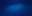# Learn how to design a trading system by Bull's Power

MetaTrader 5Trading | 17 August 2022, 11:525 9570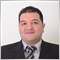### Introduction

In this new article from our series, we will learn about a new technical tool that can be used in our favor to enhance our trading. We learn about the Bull's Power indicator which is one of the technical indicators that can be used to give us insights into bulls measurement the same as we will see in this article. So, we will learn about this technical indicator in detail and we will cover this technical tool through the following topics:

We will learn what is the Bull's Power indicator, what it measures, how can calculate it manually to understand the main concept behind it, and how we can read it and this will be learned through the topic of Bull's Power definition. After understanding the basic concept of this indicator, we will learn how we can use it through some simple strategies which can be used in our favor to enhance our trading results and this will be learned through the Bull's Power strategy topic. After that, we will design a step-by-step blueprint for every strategy to help us to create a trading system for every strategy and this will be learned through the topic of Bull's Power strategy blueprint. Then, we will create a trading system for every mentioned strategy to help us to automate our signals by executing them in the MetaTrader 5 trading platform.

We will use the MetaTrader 5 trading terminal and we will write our codes by the MetaQuotes Language 5 (MQL5). If you want to learn how to download MetaTrader 5 and use MQL5, you can read the topic of Writing MQL5 code in MetaEditor from a previous article to learn more about that. I advise you to apply what you read by yourself if you want to improve your coding skill and understand it well.

The importance of programming increases day after day and this case creates the importance to learn this important tool. This importance of programming comes from the benefits of programming and they are a lot. The most important benefits of programming in trading are that it helps us to save our time as we code or create a program to do what we continuously doing, it gives us more accurate results the same as we need, and it helps us to avoid emotions which can harm our trading results.

Disclaimer: All information provided 'as is' only for educational purposes and is not prepared for trading purposes or advice. The information does not guarantee any kind of result. If you choose to use these materials on any of your trading accounts, you will do that at your own risk and you will be the only responsible.

### Bull's Power definition

In this topic, we will identify the Bull's Power indicator in detail to understand the main concept behind it. The Bull's Power indicator is the opposite of the Bear's Power that we mentioned before in a previous article Learn how to design a trading system by Bear's Power. The Bull's Power is developed by Alexander Elder, it measures the bullishness in the market and it can also give an indication if the bears come to the game or not by seeing the bulls become weak. It is an oscillator indicator that oscillates above or below zero level. So, this indicator also gives us an important insight into the most important factor in the market that makes the market move and this factor is demand and supply.

How we can calculate this indicator manually, is what we will identify in the following steps:

• Get the high (the highest values during a specific period).
• Get the exponential moving average EMA.
• Get the difference between the high and the exponential moving average.

Bull's Power = high - EMA

When bulls control the market, they keep pushing the market to higher values most of the time. So, we use the high's value in the formula to calculate the Bull's Power because we need to measure these bulls then we will get the difference between this high and the EMA to get an oscillator indicator that oscillates around zero level as we mentioned before and when we find that the value of bull's power approaches the zero level and became lower than before we can get an indication that bulls became weaker.

It is good to use this indicator with another trending indicator as it will give more effective insights the same as we will do in one of the mentioned strategies in this article. This concept is very helpful and one of the useful features of the technical analysis as we can use many concepts to get more insights and see the instrument from different perspectives and this gives us more weight to our decision.

Nowadays, we do not need to calculate this indicator manually as we have it in the MetaTrader 5 trading terminal and all we need to do is to insert it into the chart by pressing Insert --> Indicators --> Oscillators --> Bulls Power. We can see that also through the following picture: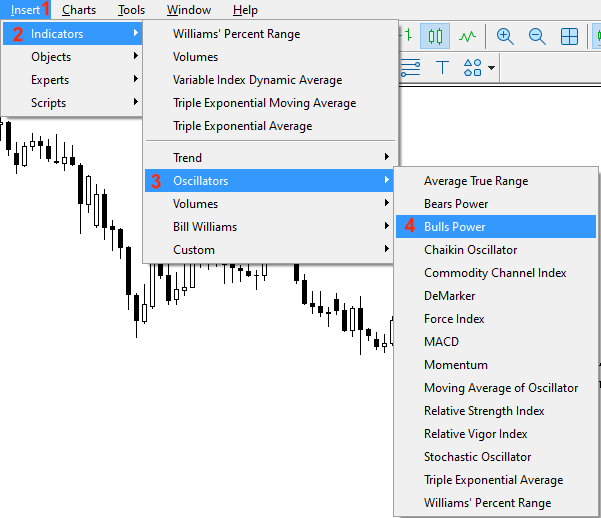After choosing the indicator, we will see the following window of its parameters: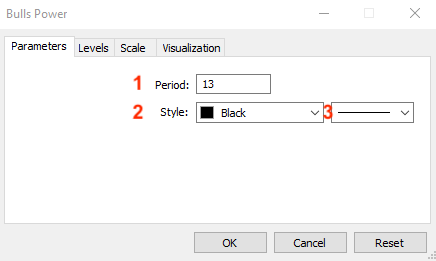1 - To determine the period that will be used in the calculation.

2 - To determine the color of bars of Bull's Power.

3 - To determine the thickness of bars of Bull's Power.

After determining these parameters and pressing "OK", we will find that the indicator is inserted into the chart the same as the following: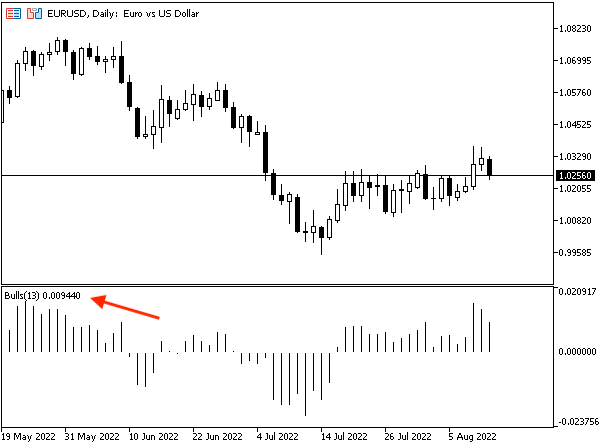As we can see in the previous chart in the lower part that we have the Bull's Power indicator is attached to the chart and its bars oscillate around zero the same as we mentioned when we find the value above zero it means that bulls control and when it approaches zero and became lower than it, this means that they became weaker. When the bulls became weaker, we can see that bears came to the game by controlling the market by pushing the price toward low levels, or at least, the market is balanced through the balance between bulls and bears.

### Bull's Power strategy

In this part, we will learn how we can use Bull's Power through simple strategies that can be used based on the basic concept of this indicator. The following are for these strategies and their conditions. I need to confirm here, that these strategies for education only as the main objective is to understand the main concept behind the indicator and how we can use them in our favor, so you must test any of them before using them on your real account to make sure that it will be good for your trading as there is no strategy is suitable for everyone.

• Strategy one: Bull's Power Movement

Based on this strategy, we need to get signals based on the position of current and previous bull's power values. If the current bull's power value is greater than the previous one, we will consider it as a signal of the rising of the Bull's Power indicator. Vice versa, if the current value is lower than the previous one, we will consider that as a signal of declining Bull's Power.

To simplify that, it will be the same as the following:

Current Bull's Power > Previous Bull's Power --> Bull's Power is Rising

Current Bull's Power < Previous Bull's Power --> Bull's Power is declining

• Strategy two: Bull's Power - Strong or Divergence

Based on this strategy, we want to get a signal that informs us if there is a strong movement or there is a divergence by evaluating four values and these values are current high, the previous high, bull power, and previous bull power. If the current high is higher than the previous high and the current bull power value is higher than the previous one, we will consider that as a signal of a strong move. In the other case, if the current high is higher than the previous high and the current bull value is lower than the previous one, we will consider that as a signal of bearish divergence.

To simplify that, it will be the same as the following:

Current high > previous high and current bull's power > previous bull's power --> strong move

Current high < previous high and current bull's power > previous bull's power --> bearish divergence

• Strategy three: Bull's Power signals

Based on this strategy, we need a signal that can be used to get buy and sell signals and we will evaluate four values to do that based on this strategy. These four values are current bull's power, zero level, current close value, and current exponential moving average. If the current bull's power is lower than the zero level and the current close is lower than the exponential moving average, we will consider it as a signal of selling. If the current bull's power is greater than the zero level and the current close is greater than the exponential moving average, this will be a signal of buying.

To simplify that, it will be the same as the following:

Current bull's power < zero level and current close < EMA --> sell

Current bear's power > zero level and current close > EMA --> buy

### Bull's Power strategy blueprint

In this topic, we will design a step-by-step blueprint for every mentioned strategy to help us to create our trading system easily and smoothly after organizing our ideas.

• Strategy one: Bull's Power Movement

We need the computer to check two values for every tick, and that will be after creating these values for sure, these values are current Bull's Power and previous Bull's Power. We need the program to check these values to know the position of everyone. If the current bull's power is greater than the previous bull's power, we need the program or the expert to return a signal of comment on the chart with the following values and each one in a separate line:

• Bull's Power is rising
• Bull's Power Value
• Bull's Power Previous Value

There is another scenario that we need to consider. If the current Bull's Power value is lower than the previous one, we need the expert advisor to return a comment also with the following values and each value in a separate line:

• Bull's Power is declining
• Bull's Power Value
• Bull's Power Previous Value.

The following is the simple blueprint for this trading system based on its strategy: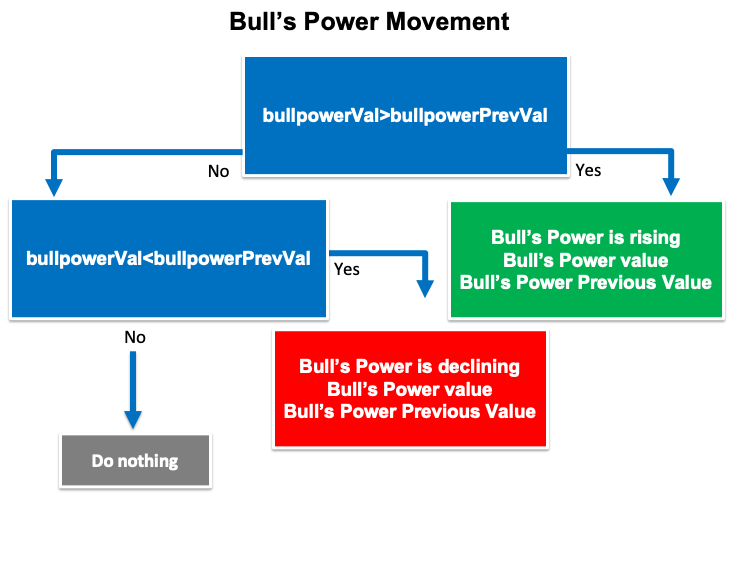• Strategy two: Bull's Power- Strong or Divergence

According to this strategy, we need the trading system to check four values and these values are current high, previous high, current bull power, and previous bull power values. After that, we need the trading system to decide if the current high value is higher than the previous one and at the same time if the current bull's power value is higher than the previous one, we need the trading system to return a signal as a comment on the chart with the following values and each one of them will be in a separate line:

• Strong Move
• Current High Value
• Previous High Value
• Current Bull's Power Value
• Previous Bull's Power Value

In the other case, if the current high value is higher than the previous one and at the same time, the current bull's power value is lower than the previous one, we need the trading system to return a signal of comment on the chart with the following values and each value in a separate line:

• Bearish divergence
• Current High Value
• Previous High Value
• Current Bull's Power Value
• Previous Bull's Power Value

The following is the blueprint in a simple form visually to help us to create a trading system based on this strategy.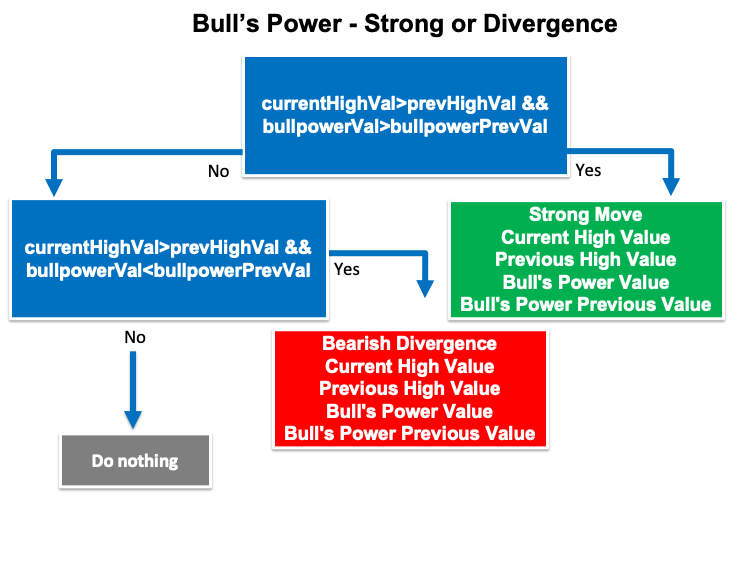• Strategy three: Bull's Power signals

According to this strategy, we need to create a trading system that checks four values for every tick and these values are the current bull's power, the zero level, the current close, and the current exponential moving average. Then we need to decide if the current bull's power is lower than the zero level and at the same time if the current close is lower than the exponential moving average, we need the expert advisor to return a signal of comment on the chart with the following values and each one of them in a separate line:

• Sell Signal
• Current Close Value
• Current EMA Value
• Current Bull's Power Value

In the other case, if the current bull's power is greater than zero and at the same time, the current close is greater than the exponential moving average, we need the trading system to return a signal of comment with the following values, each value will be in a separate line:

• Current Close Value
• Current EMA Value
• Current Bull's Power Value

The following is a simple step-by-step blueprint to organize our ideas to create a trading system based on this strategy.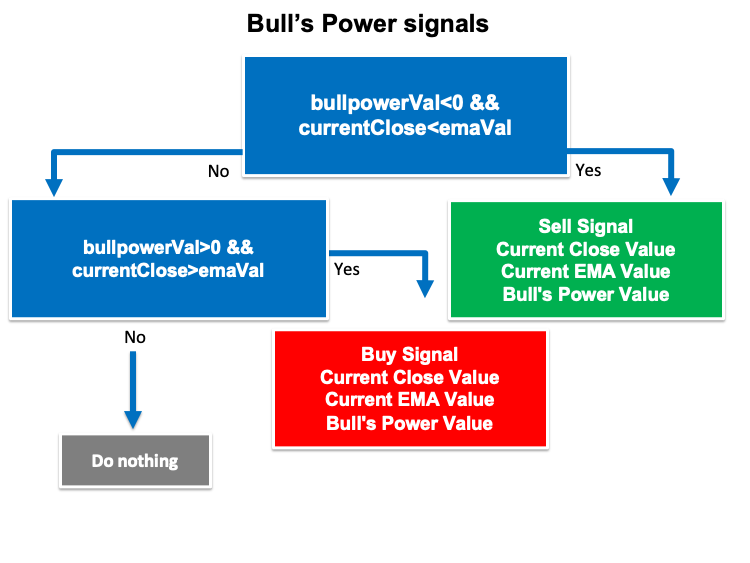### Bull's Power trading system

In this topic, we will learn how to create a trading system for each mentioned strategy but first we will create a simple trading system that can be used to generate a comment with the current Bull's Power value on the chart automatically to be used as a base for all strategies.

The following is the code of how to create this trading system:

Step one: We will create an array for the "bullpower" by using the double function:

`double bullpowerArray[];`

Step two: we will sort this created array by using the "ArraySetAsSeries" function to return a boolean value.

`ArraySetAsSeries(bullpowerArray,true);`

Step three: We will create an integer variable for "bullpowerDef", we will define the bull power indicator by using the "iBullsPower" function to return the handle of the indicator. Parameters are:

symbol: we will use (_Symbol) to be applied to the current symbol.

period: we will use (_Period) to be applied to the current period or timeframe.

ma_period: we will use 13 for the period of the usable moving average.

`int bullpowerDef = iBullsPower(_Symbol,_Period,13);`

Step four: We will fill the created array by using the "CopyBuffer" function to get the data from the Bull's Power indicator. Parameters of this function:

indicator_handle: for indicator handle and we will use (bullpowerDef).

buffer_num: for indicator buffer number and we will use (0).

start_pos: for start position and we will use (0).

count: for the amount to copy and we will use (3).

buffer[]: for the target array to copy and we will use (bullpowerArray).

`CopyBuffer(bullpowerDef,0,0,3,bullpowerArray);`

Step five: We will get the "bullpowerVal" after creating a variable by using the "NormalizeDouble" function to return the double type value. Parameters of this function:

value: we will use (bullpowerArray) as a normalized number.

digits: we will use (6) as a number of digits after the decimal point.

`double bullpowerVal = NormalizeDouble(bullpowerArray,6);`

Step six: we will use a comment function to generate a comment on the chart with the current bull power value:

`Comment("Bull's Power Value is ",bullpowerVal);`

The following is the full code of the previous steps:

```//+------------------------------------------------------------------+
//|                                          Simple Bull's Power.mq5 |
//|                                  Copyright 2022, MetaQuotes Ltd. |
//|                                             https://www.mql5.com |
//+------------------------------------------------------------------+
#property version   "1.00"
//+------------------------------------------------------------------+
void OnTick()
{
double bullpowerArray[];

ArraySetAsSeries(bullpowerArray,true);

int bullpowerDef = iBullsPower(_Symbol,_Period,13);

CopyBuffer(bullpowerDef,0,0,3,bullpowerArray);

double bullpowerVal = NormalizeDouble(bullpowerArray,6);

Comment("Bull's Power Value is ",bullpowerVal);

}
//+------------------------------------------------------------------+```

After that we compile this code to see the expert of this code in the navigator window the same as the following: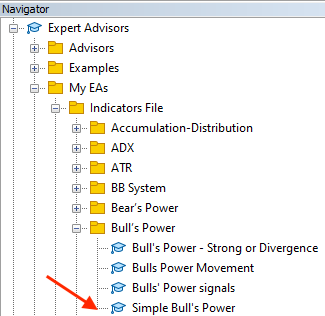By double-clicking on this the Simple Bull's Power to execute it on the MetaTrader 5 terminal, we will see its window the same as the following: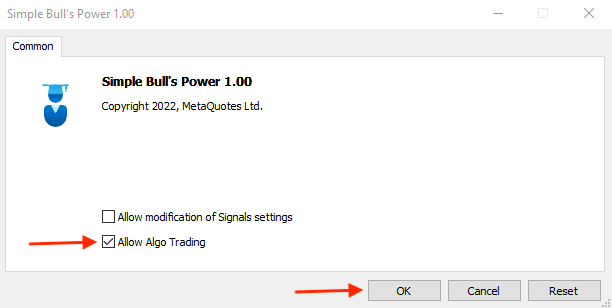After pressing "OK", we will find the expert is attached to the chart the same as the following: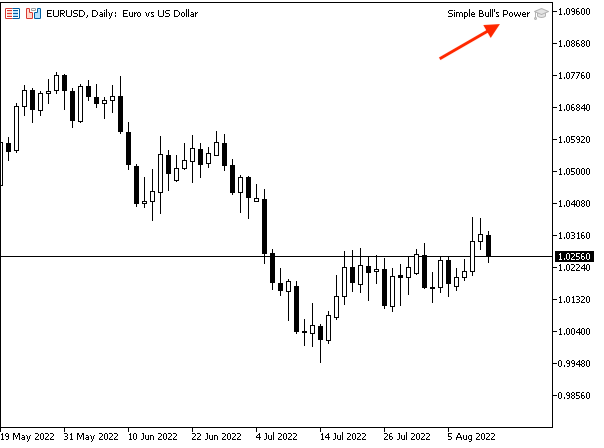As we can see on the previous chart in the top right corner that the expert is already attached to chart. Now, we are ready to receive the automatic comment with the updated current bull power value on the chart the same as the following example from testing: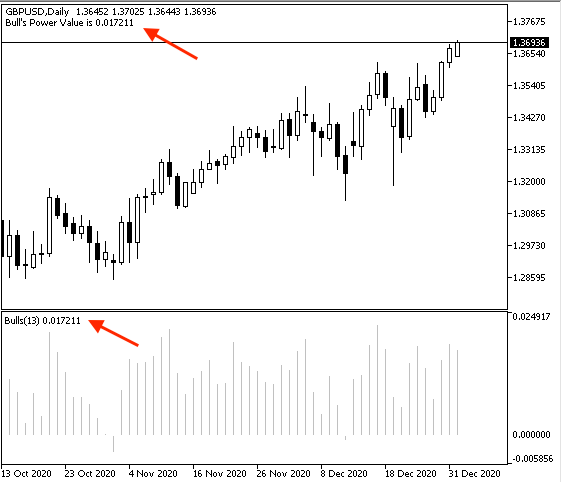As we can see on the previous chart in the top left corner that we have the current bull power value a comment.

• Strategy one: Bull's Power Movement

The following is the full code to create a trading system based on this strategy:

```//+------------------------------------------------------------------+
//|                                         Bulls Power Movement.mq5 |
//|                                  Copyright 2022, MetaQuotes Ltd. |
//|                                             https://www.mql5.com |
//+------------------------------------------------------------------+
#property version   "1.00"
//+------------------------------------------------------------------+
void OnTick()
{
double bullpowerArray[];

ArraySetAsSeries(bullpowerArray,true);

int bullpowerDef = iBullsPower(_Symbol,_Period,13);

CopyBuffer(bullpowerDef,0,0,3,bullpowerArray);

double bullpowerVal = NormalizeDouble(bullpowerArray,6);
double bullpowerPrevVal = NormalizeDouble(bullpowerArray,6);

if(bullpowerVal>bullpowerPrevVal)
{
Comment("Bull's Power is rising","\n",
"Bull's Power Value is ",bullpowerVal,"\n",
"Bull's Power Previous Value is ",bullpowerPrevVal);
}

if(bullpowerVal<bullpowerPrevVal)
{
Comment("Bull's Power is declining","\n",
"Bull's Power Value is ",bullpowerVal,"\n",
"Bull's Power Previous Value is ",bullpowerPrevVal);
}
}
//+------------------------------------------------------------------+```

Differences in this code the same as the following:

Defining the current bull's power value "bullpowerVal" and the previous bull's power value "bullpowerPrevVal":

```   double bullpowerVal = NormalizeDouble(bullpowerArray,6);
double bullpowerPrevVal = NormalizeDouble(bullpowerArray,6);```

Conditions of the strategy:

In case of rising:

```   if(bullpowerVal>bullpowerPrevVal)
{
Comment("Bull's Power is rising","\n",
"Bull's Power Value is ",bullpowerVal,"\n",
"Bull's Power Previous Value is ",bullpowerPrevVal);
}```

In case of declining:

```   if(bullpowerVal<bullpowerPrevVal)
{
Comment("Bull's Power is declining","\n",
"Bull's Power Value is ",bullpowerVal,"\n",
"Bull's Power Previous Value is ",bullpowerPrevVal);
}```

After compiling we will find the expert in the navigator window: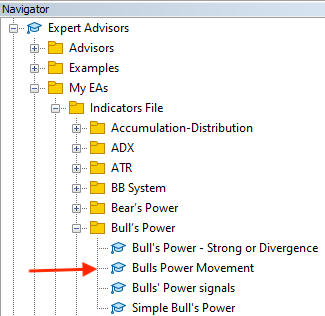By dragging and dropping it on the desired chart, we will find the window of it the same as the following: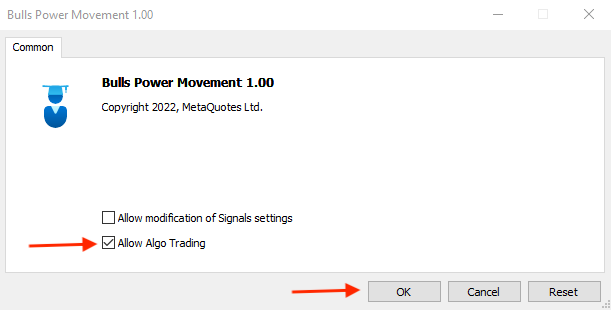After pressing "OK", we will find the expert is attached to the chart: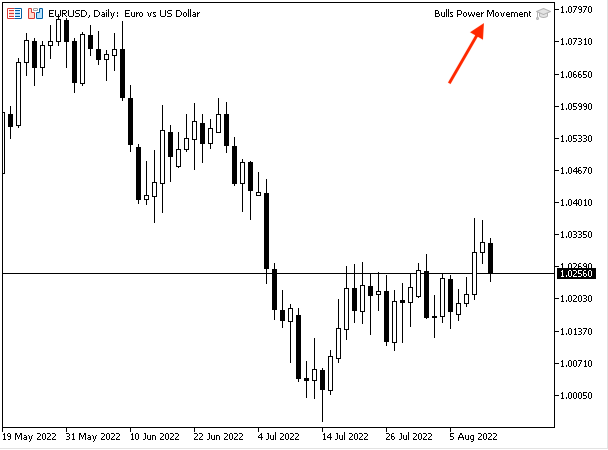As we can see in the previous chart in the top right corner from the chart that we have the expert is already attached to the chart and now we are ready to get its signals the same as the following.

In case of rising with current data: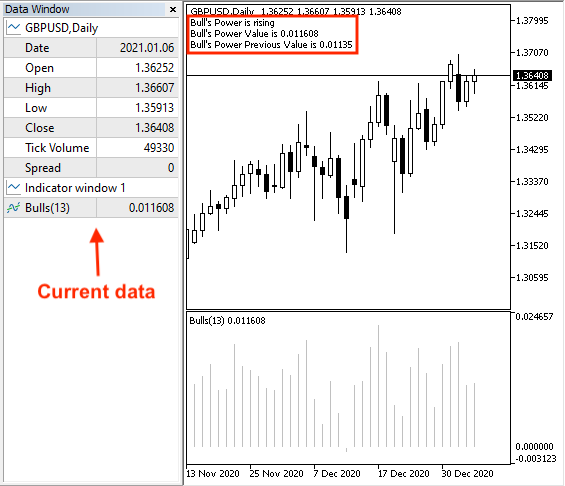As we can see on the previous chart in the upper part of the chart, we have generated a rising signal, the current bull's power value, and the previous bull's power value. In the data window, we can find the current value of the bull's power.

In case of rising with previous data: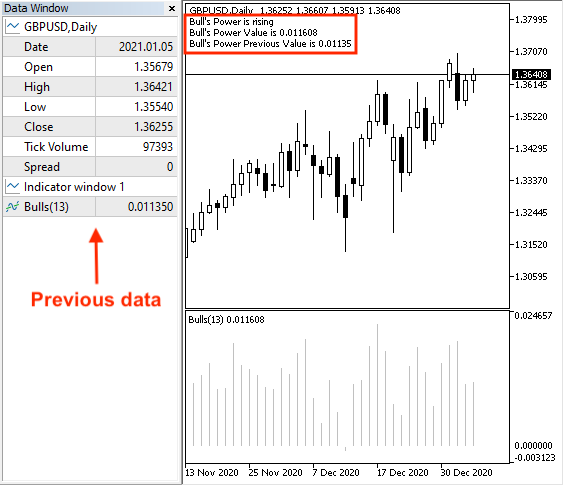As we can see on the previous chart, we can find only the difference in the data window as we can find the previous value of the bulls power.

In case of declining with current data: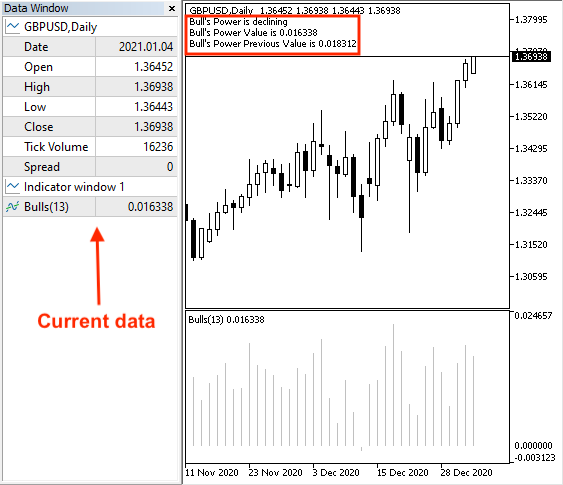As we can see on the previous chart in the upper part of the chart, we have generated a declining signal, the current bull's power value, and the previous bull's power value. In the data window, we can find the current value of the bull's power.

In case of declining with previous data: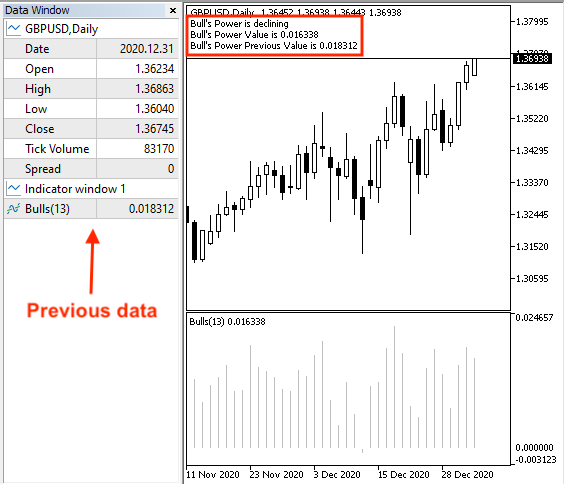As we can see on the previous chart, we can find the only difference in the data window as we can find the last value of the bull's power.

• Strategy two: Bull’s Power - Strong or Divergence

The following is the full code to create a trading system based on this strategy:

```//+------------------------------------------------------------------+
//|                          Bull's Power - Strong or Divergence.mq5 |
//|                                  Copyright 2022, MetaQuotes Ltd. |
//|                                             https://www.mql5.com |
//+------------------------------------------------------------------+
#property version   "1.00"
//+------------------------------------------------------------------+
void OnTick()
{
double bullpowerArray[];
MqlRates priceArray[];

ArraySetAsSeries(bullpowerArray,true);
int Data=CopyRates(_Symbol,_Period,0,3,priceArray);

int bullpowerDef = iBullsPower(_Symbol,_Period,13);

CopyBuffer(bullpowerDef,0,0,3,bullpowerArray);

double bullpowerVal = NormalizeDouble(bullpowerArray,6);
double bullpowerPrevVal = NormalizeDouble(bullpowerArray,6);

double currentHighVal=NormalizeDouble(priceArray.high,6);
double prevHighVal=NormalizeDouble(priceArray.high,6);

if(currentHighVal>prevHighVal && bullpowerVal>bullpowerPrevVal)
{
Comment("Strong Move","\n",
"Current High Value is ",currentHighVal,"\n",
"Previous High Value is ",prevHighVal,"\n",
"Bull's Power Value is ",bullpowerVal,"\n",
"Bull's Power Previous Value is ",bullpowerPrevVal);
}

if(currentHighVal>prevHighVal && bullpowerVal<bullpowerPrevVal)
{
Comment("Bearish divergence","\n",
"Current High Value is ",currentHighVal,"\n",
"Previous High Value is ",prevHighVal,"\n",
"Bull's Power Value is ",bullpowerVal,"\n",
"Bull's Power Previous Value is ",bullpowerPrevVal);
}
}
//+------------------------------------------------------------------+```

Differences in this code will be the same as the following:

Creating two arrays, bullpowerArray and priceArray:

```   double bullpowerArray[];
MqlRates priceArray[];```

Sorting these created arrays, for the bullpowerArray we will use using the "ArraySetAsSeries" function the same as we learned before. For the priceArray, we will use the "CopyRates" function to get historical data of "MqlRates" and its parameters are:

• symbol name: we will use (_Symbol)
• timeframe: we will use (_Period)
• start time: we will use (0)
• stop time: we will use (3)
• rates array: we will use (priceArray)
```   ArraySetAsSeries(bullpowerArray,true);
int Data=CopyRates(_Symbol,_Period,0,3,priceArray);```

Defining current and previous values of bull's power and highs by using the "NormalizeDouble" function.

```   double bullpowerVal = NormalizeDouble(bullpowerArray,6);
double bullpowerPrevVal = NormalizeDouble(bullpowerArray,6);

double currentHighVal=NormalizeDouble(priceArray.high,6);
double prevHighVal=NormalizeDouble(priceArray.high,6);```

Conditions of strategy:

In case of strong move:

```   if(currentHighVal>prevHighVal && bullpowerVal>bullpowerPrevVal)
{
Comment("Strong Move","\n",
"Current High Value is ",currentHighVal,"\n",
"Previous High Value is ",prevHighVal,"\n",
"Bull's Power Value is ",bullpowerVal,"\n",
"Bull's Power Previous Value is ",bullpowerPrevVal);
}```

In case of bearish divergence:

```   if(currentHighVal>prevHighVal && bullpowerVal<bullpowerPrevVal)
{
Comment("Bearish divergence","\n",
"Current High Value is ",currentHighVal,"\n",
"Previous High Value is ",prevHighVal,"\n",
"Bull's Power Value is ",bullpowerVal,"\n",
"Bull's Power Previous Value is ",bullpowerPrevVal);
}```

After compiling this code we can find the expert of this trading system in the navigator window: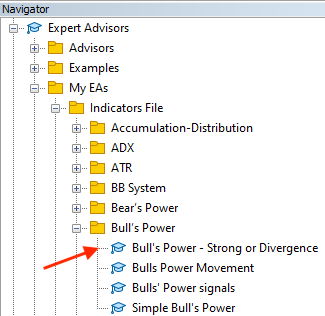By double-clicking this expert for execution, we will find its window the same as the following: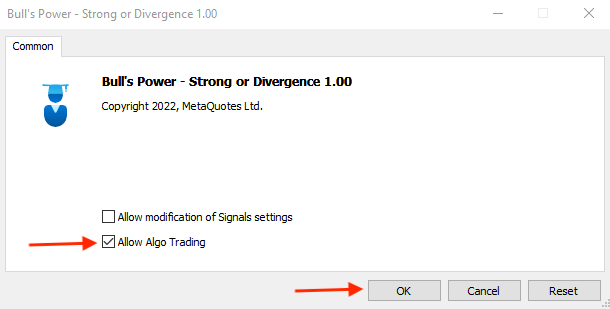After pressing "OK", we will find the expert is attached the same as the following: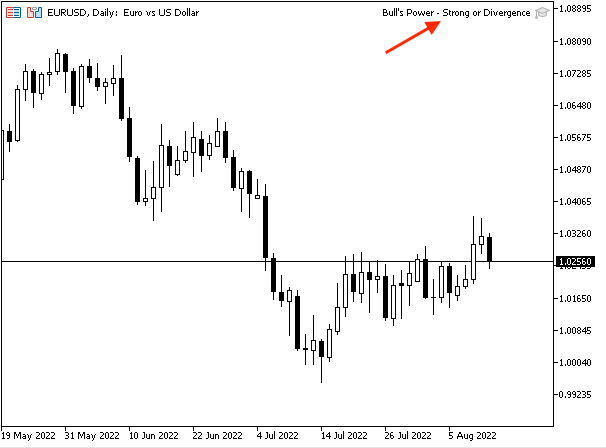As we can see in the top right corner that the expert is attached and we're ready to receive its signals the same as the following.

In case of a strong move with current data: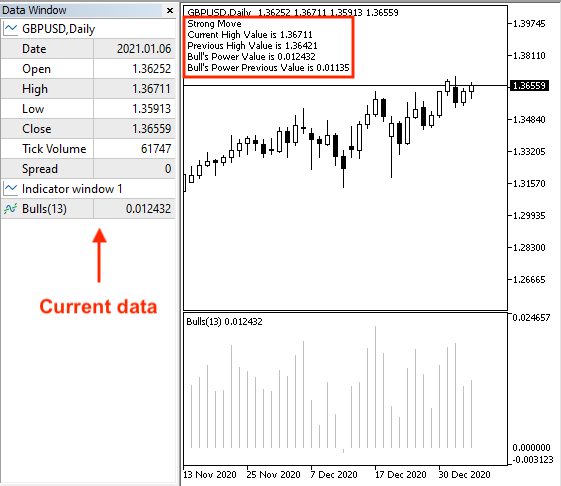As we can see on the chart we have the desired signal the same as we want based on this strategy with a comment of the following values:

• Strong move
• Current high value
• Previous high value
• Current Bull's Power value
• Previous Bull's Power value

In case of strong with previous data: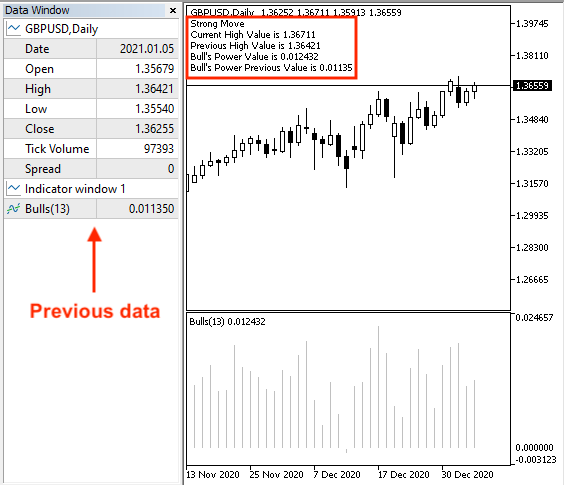As we can see on the previous chart we have the same comment as a signal on the chart with the same values but the difference is the previous data window.

In case of bearish divergence with current data: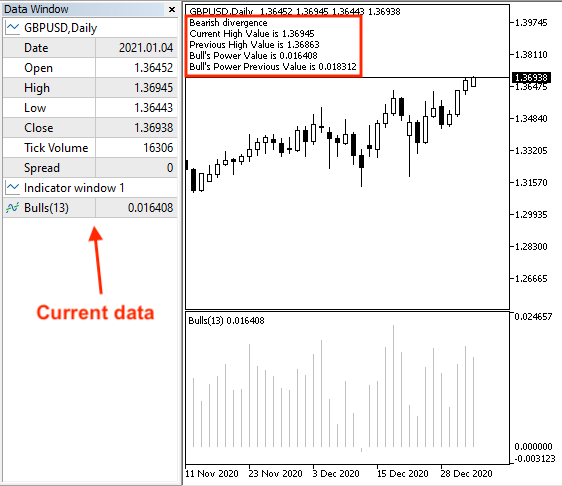As we can see on the previous chart we have a comment on the chart as a comment with the following values:

• Bearish Divergence
• Current high value
• Previous high value
• Current Bull's Power value
• Previous Bull's Power value

In case of bearish divergence with previous data:As we can see on the previous chart we have a comment on the chart with the same value but here we can find the previous data window.

• Strategy three: Bull's Power signals

The following is the full code to create a trading system based on this strategy.

```//+------------------------------------------------------------------+
//|                                         Bulls' Power signals.mq5 |
//|                                  Copyright 2022, MetaQuotes Ltd. |
//|                                             https://www.mql5.com |
//+------------------------------------------------------------------+
#property version   "1.00"
//+------------------------------------------------------------------+
void OnTick()
{
double bullpowerArray[];
double maArray[];
MqlRates priceArray[];

ArraySetAsSeries(bullpowerArray,true);
ArraySetAsSeries(maArray,true);
int Data=CopyRates(_Symbol,_Period,0,3,priceArray);

int bullpowerDef = iBullsPower(_Symbol,_Period,13);
int maDef = iMA(_Symbol,_Period,13,0,MODE_EMA,PRICE_CLOSE);

CopyBuffer(bullpowerDef,0,0,3,bullpowerArray);

double bullpowerVal = NormalizeDouble(bullpowerArray,6);

double emaVal = NormalizeDouble(maArray,6);

double currentClose=NormalizeDouble(priceArray.close,6);

if(bullpowerVal<0 && currentClose<emaVal)
{
Comment("Sell Signal","\n",
"Current Close Value is ",currentClose,"\n",
"Current EMA Value is ",emaVal,"\n",
"Bull's Power Value is ",bullpowerVal);
}

if(bullpowerVal>0 && currentClose>emaVal)
{
"Current Close Value is ",currentClose,"\n",
"Current EMA Value is ",emaVal,"\n",
"Bull's Power Value is ",bullpowerVal);
}
}
//+------------------------------------------------------------------+```

Differences in this code:

Creating three arrays, bullpowerArray, maArray, and priceArray.

```   double bullpowerArray[];
double maArray[];
MqlRates priceArray[];```

Sorting these three created arrays.

```   ArraySetAsSeries(bullpowerArray,true);
ArraySetAsSeries(maArray,true);
int Data=CopyRates(_Symbol,_Period,0,3,priceArray);```

We will define the Bull's Power indicator by using the "iBullsPower" the same as we mentioned before after creating an integer variable for the "bullpowerDef" and the Moving Average indicator by using the "iMA" function that returns the handle of the moving average indicator after creating an integer variable for the "maDef".

```   int bullpowerDef = iBullsPower(_Symbol,_Period,13);
int maDef = iMA(_Symbol,_Period,13,0,MODE_EMA,PRICE_CLOSE);```

Filling the array by using the "CopyBuffer" function to get the data from the Bull's Power and moving average indicators.

```   CopyBuffer(bullpowerDef,0,0,3,bullpowerArray);

We will define the current bull's power, exponential moving average, and current closing price.

```   double bullpowerVal = NormalizeDouble(bullpowerArray,6);
double emaVal = NormalizeDouble(maArray,6);
double currentClose=NormalizeDouble(priceArray.close,6);```

Conditions of this strategy:

In case of the sell signal

```   if(bullpowerVal<0 && currentClose<emaVal)
{
Comment("Sell Signal","\n",
"Current Close Value is ",currentClose,"\n",
"Current EMA Value is ",emaVal,"\n",
"Bull's Power Value is ",bullpowerVal);
}```

In case of the buy signal:

```   if(bullpowerVal>0 && currentClose>emaVal)
{
"Current Close Value is ",currentClose,"\n",
"Current EMA Value is ",emaVal,"\n",
"Bull's Power Value is ",bullpowerVal);
}```

After compiling this code, we will find the expert in the navigator window the same as the following: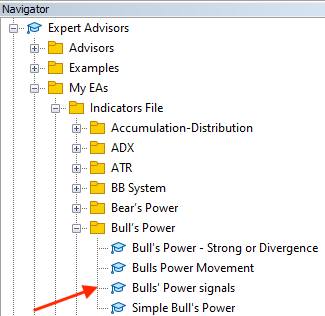By double-clicking the expert to be executed on the chart, we will find its window the same as the following: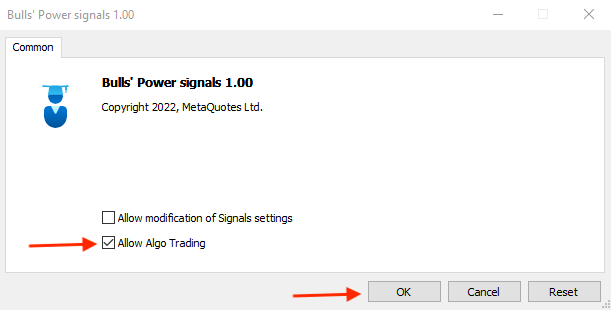After pressing "OK", we will find that the expert is attached to the chart the same as the following: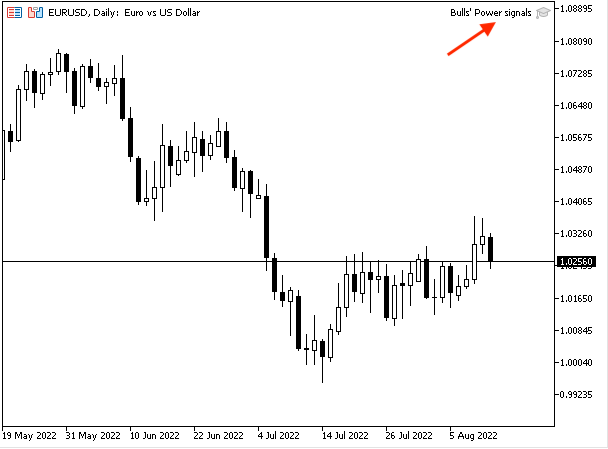We can see the expert of Bull's Power signals is attached to the chart in the top right corner. Then, we're ready to receive signals based on this trading strategy the same as the following:

In case of the sell signal: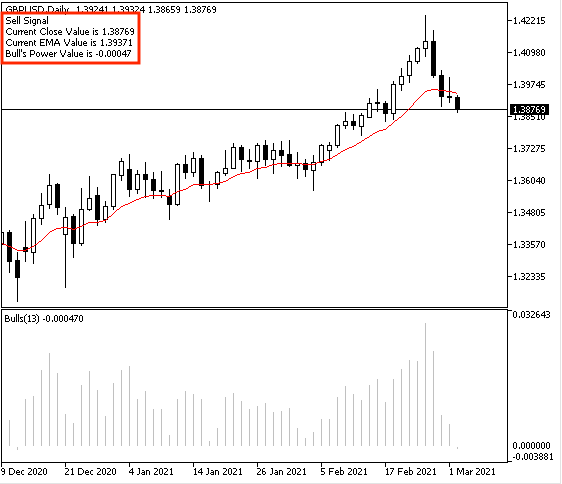As we can see on the chart we have a comment with the following values:

• Sell signal
• Current close value
• Current EMA value
• Bull's Power value

In case of buy signal: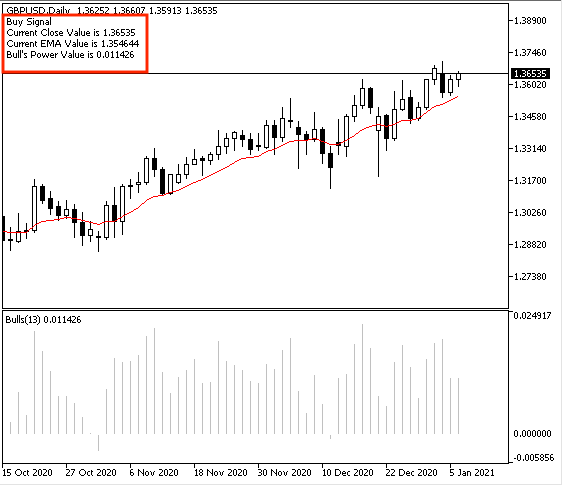As we can see on the chart the desired signal as a comment on the chart with the following values:

• Current close value
• Current EMA value
• Bull's Power value

By the previous, we created a trading system for each mentioned strategy to generate automated signals.

### Conclusion

I hope that we covered this indicator through the previous topics. We learned in more detail what is the Bull's Power indicator, what it measures, how we can calculate it, how we can read it, and how we can insert and use the MetaTrader 5 built-in Bull's Power indicator through the topic of Bull's Power definition. After that, we learned how to use this indicator based on the basic concept behind it through some mentioned simple strategies, and the basic objective of these strategies is to learn how we can use this indicator and you must test them before using them on your real account to make sure that they are suitable for your trading style to generate profits by them. These strategies were the same as the following:

• Bull's Power Movement: this strategy can be used to determine the direction of the Bull's Power indicator if it is rising or declining.
• Bull's Power Strong or divergence: it can be used to determine if we have a strong move or bearish divergence.
• Bull's Power signals: it can be used to give us buy or sell signals.

After that, we designed a step-by-step blueprint for every mentioned strategy to help us to create a trading system smoothly after organizing our ideas through these blueprints. Then, we learned how to create a trading system for every mentioned strategy to get signals automatically after coding them on MQL5 to be executed on the MetaTrader 5 trading terminal. I hope that you applied and tried to write mentioned codes by yourself to improve your coding skills if you want and this will enhance and improve your learning curve as a programmer.

I hope also that you find this article useful for you to enhance your trading and improve your trading results. If you want to read more similar articles about learning how to design a trading system based on the most popular technical indicators you can read my other articles in this series as we have many technical indicators that we shared in these articles and designed trading systems based on them and based on the basic concept behind them through simple strategies.

Attached files |

#### Other articles by this author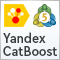Metamodels in machine learning and trading: Original timing of trading orders
Metamodels in machine learning: Auto creation of trading systems with little or no human intervention — The model decides when and how to trade on its own.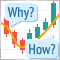Learn how to design a trading system by Bear's Power
Welcome to a new article in our series about learning how to design a trading system by the most popular technical indicator here is a new article about learning how to design a trading system by Bear's Power technical indicator.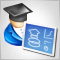Developing a trading Expert Advisor from scratch (Part 19): New order system (II)
In this article, we will develop a graphical order system of the "look what happens" type. Please note that we are not starting from scratch this time, but we will modify the existing system by adding more objects and events on the chart of the asset we are trading.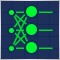Data Science and Machine Learning — Neural Network (Part 01): Feed Forward Neural Network demystified
Many people love them but a few understand the whole operations behind Neural Networks. In this article I will try to explain everything that goes behind closed doors of a feed-forward multi-layer perception in plain English.